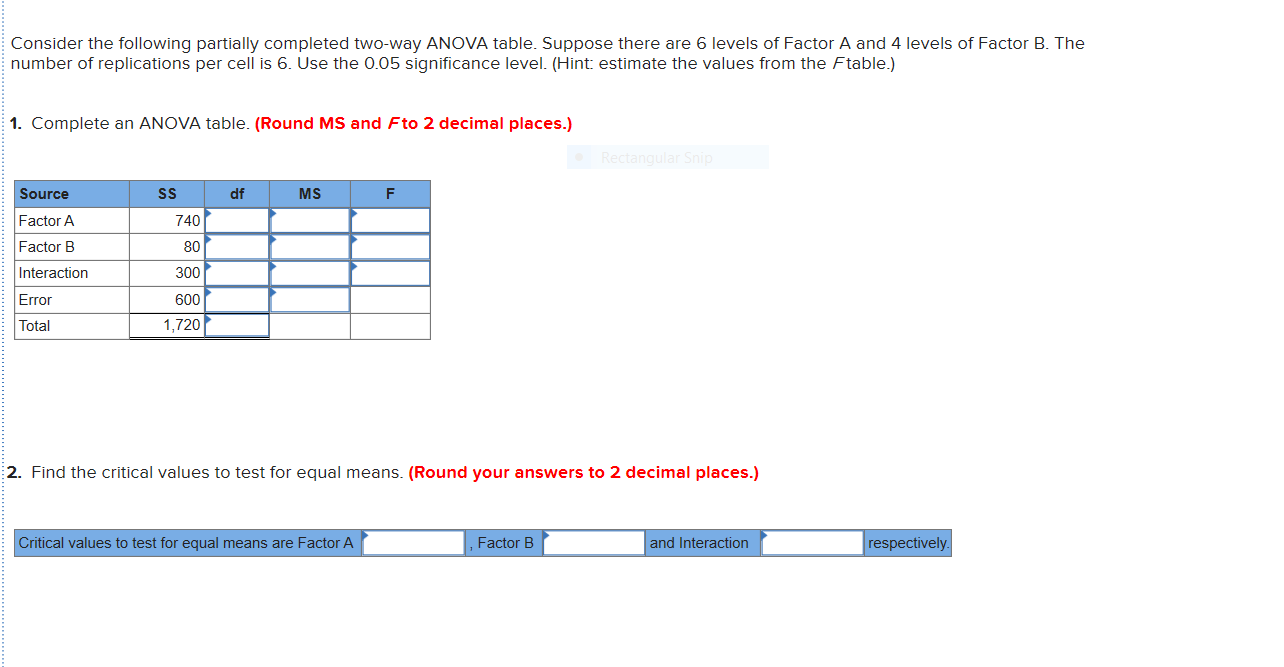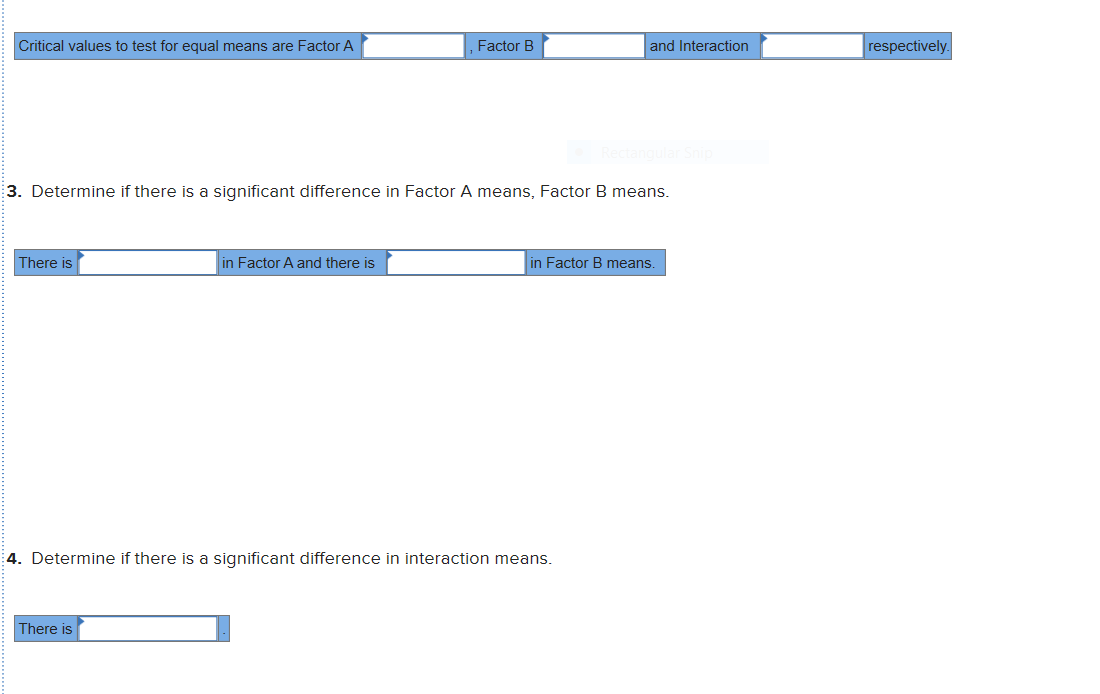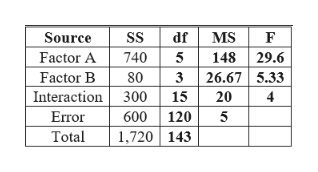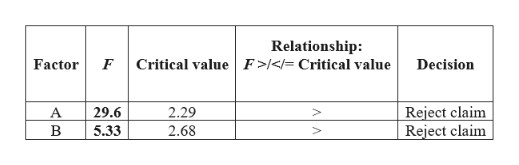# Consider the following partially completed two-way ANOVA table. Suppose there are 6 levels of Factor A and 4 levels of Factor B. Thenumber of replications per cell is 6. Use the 0.05 significance level. (Hint: estimate the values from the Ftable.)1. Complete an ANOVA table. (Round MS and Fto 2 decimal places.)Rectangular SnipSourceSsdfMSFactor A740Factor B80Interaction300Error600Total1,7202. Find the critical values to test for equal means. (Round your answers to 2 decimal places.)Factor Band InteractionCritical values to test for equal means are Factor Arespectively respectivelyand InteractionCritical values to test for equal means are Factor AFactor B3. Determine if there is a significant difference in Factor A means, Factor B means.in Factor B means.There isin Factor A and there is4. Determine if there is a significant difference in interaction means.There is

Questionhelp_outlineImage TranscriptioncloseConsider the following partially completed two-way ANOVA table. Suppose there are 6 levels of Factor A and 4 levels of Factor B. The number of replications per cell is 6. Use the 0.05 significance level. (Hint: estimate the values from the Ftable.) 1. Complete an ANOVA table. (Round MS and Fto 2 decimal places.) Rectangular Snip Source Ss df MS Factor A 740 Factor B 80 Interaction 300 Error 600 Total 1,720 2. Find the critical values to test for equal means. (Round your answers to 2 decimal places.) Factor B and Interaction Critical values to test for equal means are Factor A respectively fullscreenhelp_outlineImage Transcriptioncloserespectively and Interaction Critical values to test for equal means are Factor A Factor B 3. Determine if there is a significant difference in Factor A means, Factor B means. in Factor B means. There is in Factor A and there is 4. Determine if there is a significant difference in interaction means. There is fullscreen
check_circleExpert Solution
Step 1

Note:

Hi there! Thank you for posting the question. As your question has more than 3 parts, we have solved the first 3 for you, according to our policy. If you need help with any other part, please re-post the question and mention the part you need help with.

Step 2

Explanation:

Part (1):

Here, the number of levels of Factor A is, k1 = 6.

The number of levels of Factor B is, k2 = 4.

The number of replications per cell is, r = 6.

Thus, the degrees of freedom (df) for Factor A is, k1 – 1 = 5; the df for Factor B is, k2 – 1 = 3.

Since Interaction = Factor A × Factor B, df for Interaction is 5 × 3 = 15.

Since there are 6 levels of Factor A and 4 levels of Factor B, there are a total of 6 × 4 = 24 cells. Each cell has 6 replicates. So, total number of observations, n = 24 × 6 = 144. Thus, total df is, n – 1 = 143.

As a result, error df is, 143 – 5 – 3 – 15 = 120.

The MS or mean of squares is the SS or sum of squares, divided by the corresponding df.

The F for a particular factor is the MS for that factor, divided by the error MS; same for the Interaction.

Using this information, the ANOVA table is completed as follows:help_outlineImage TranscriptioncloseMS Source Factor A Factor B Interaction SS df 148 29.6 326.67 5.33 15 20 740 5 80 300 4 Error 600 120 5 1,720 143 Total fullscreen
Step 3

Parts 2 and 3:

The F-test is used for testing the significant effects of Factor A, Factor B, and Interaction.

The numerator and denominator df’s for testing the effect of Factor A are, 5, 120.

The significance level is, α = 0.05.

Thus, the critical value is, F0.05; 5, 120.

Using Excel formula, =F.INV.RT(0.05,5,120), the critical value for Factor A is 2.29.

The numerator and denominator df’s for testing the effect of Factor B are, 3, 120.

Thus, the critical value is, F0.05; 3, 120.

Using Excel formula, =F.INV.RT(0.05,3,120), the critical value for Factor A is 2.68.

The numerator and denominator df&r...help_outlineImage TranscriptioncloseRelationship: Critical value F><=Critical value Factor Decision Reject claim Reject claim 2.29 A 29.6 5.33 2.68 fullscreen

### Want to see the full answer?

See Solution

#### Want to see this answer and more?

Solutions are written by subject experts who are available 24/7. Questions are typically answered within 1 hour*

See Solution
*Response times may vary by subject and question
Tagged in

### Hypothesis Testing